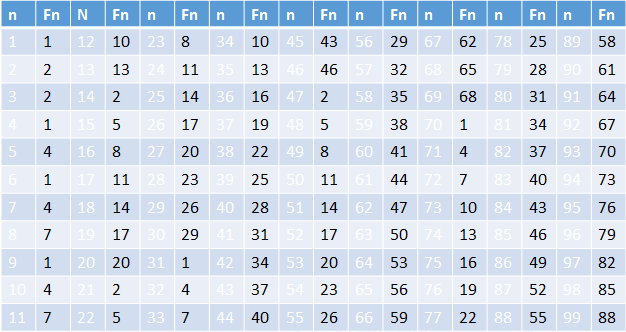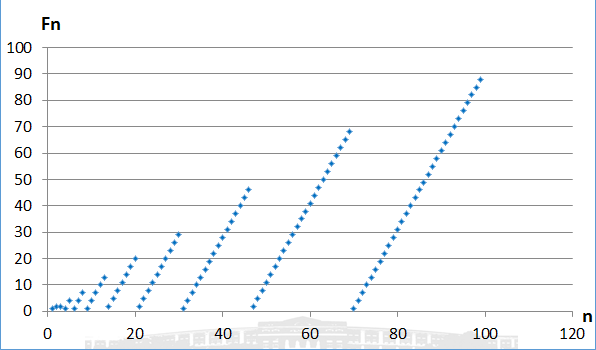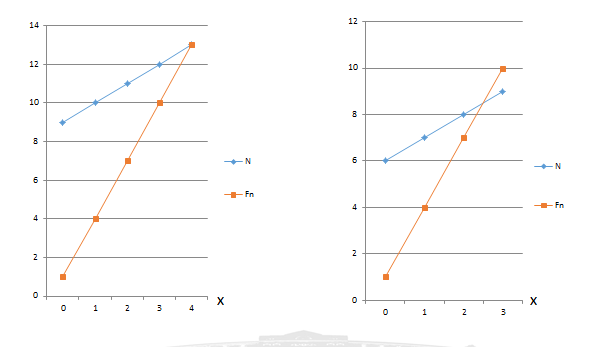# 约瑟夫环问题（数学递推法）

罗马人攻占了桥塔帕特，41个人藏在一个山洞中躲过了这场浩劫。这41个人中，包括历史学家Josephus（约瑟夫）和他的一个朋友。剩余的39个人为了表示不向罗马人屈服，决定集体自杀。大家制定了一个自杀方案，所有这41个人围成一个圆圈，由第一个人开始顺时针报数，每报数为3的人就立刻自杀，然后再由下一个人重新开始报数，仍然是每报数为3的人就立刻自杀……，直到所有的人都自杀身亡为止。约瑟夫和他的朋友并不想自杀，于是约瑟夫想到了一个计策，他们两个同样参与到自杀方案中，但是最后却躲过了自杀。

关于解约瑟夫环问题的解决方法有许多，有通过建立模型，模拟游戏规则的；有找出递归关系式，用递归实现的。不知道你们有没有看懂：F(n)与F(n-1)之间的关系式是怎么推导出来的，反正我这个考研数学刚刚过线的人只能望洋兴叹。但我并没有放弃，条条大路通罗马，此路不通另辟西路，我选的路比较简单粗暴。不是要找F(n)与F(n-1)的关系吗？我就直接一一列出1-99个人组成的环中最后胜利者的编号（m = 3），如下表所示（n 表示环中人的个数）：当我运行程序后，看到黑屏中出现这些数据时，我还是挺兴奋的，因为这些数据一眼看去，就能发现是有规律的。接下来我的工作有点类似小学生做的看数找规律：

首先，你会发现有很大一部分数是等差递增的，相邻之间相差3，正好等于我选取的m。

其次，你会发现分好几个递增梯队，当某个递增梯队中编号达到最大值后（很明显编号数不可能大于n），紧接着下一个数又会变成下个递增梯队的最小数。关键就是在这两个数之间，如果能找出它俩有什么关系，就能将所有的数理顺。

最后，我着眼于n为30、31这两个数之间。n为30时，编号为29；我就想如果n为31时，仍然还保持着这个递增，那么其编号为 29 + 3 = 32。但是这显然不符合逻辑，一共只有31个人，不会存在编号为32的。假设如果成立，编号为32，而现实中n为31时，编号为1；观察31、32和1这三个数，我想你应该能看出这三个数的关系了吧：32 % 31 = 1。

为了方便介绍，将n当做自变量，编号为因变量，函数F(n)关系式为：

F(1) = 0

F(n+1) = (F(n) + m) % (n+1)  (n > 1)

这里对F(1) = 0要重点介绍下，按照上面的表格中的数据，按道理应该是F(1) = 1，其实我一开始也是这么写的。但在后面编写代码时出现错误，这里先提前提出来，下列表（m = 5）

 n 1 2 Fn 1 2

如果按照F(1) = 1，那么F(2)=(F(1) + m) % 2 = (1+5) % 2 = 0。很明显答案不对，而且按照我从1开始编号，也不会存在编号0。这里主要是求余运算的特性造成的，从1开始编码，就永远得不到编码为2，而只会整除等于0。所以只能从0开始编码，输出编号时加1即可。

和递归的递推公式相比，似乎看上去很像，但它们之间的思想有着本质的区别。递归推导式是从上到下，不断进入递归再回溯；而我的推导式，是从下到上，符合正常的逻辑思维，比较容易理解。求解问题时，你可以假设上面的表已经存在，而你只需关心这些数据递增规律及转折处如何转折，找到解对应表格中的数据。这时我深刻体会到，似乎和约瑟夫环的规则已经完全没什么关系了，也许这就是数学高度抽象的魅力吧！具体代码如下：

#include <stdio.h>
int main()
{
int n, m, i, s = 0;
printf ("N M = ");
scanf("%d%d", &n, &m);
for (i = 2; i <= n; i++)
{
s = (s + m) % i;
}
printf ("\n The winner is %d\n", s+1);

return 0;
}


为了更直观的观察上表的数据，将N的值作为横坐标，Fn的值作为纵坐标，画出下图：从图中我们能发现，这些离散的点分成几段斜率大于0的线段（斜率 = m）。随着n的增大，越往右，线段的长度越长。 当n取值很大时，线段长度能达到100 -1000数量级。根据上面的算法，如果已知该线段的最小值Fn，求该线段的最大值，需要重复执行F(n+1) = F(n) + m语句100 – 1000次，乃至更多。这种情况显然造成了浪费，如果我们能求出该线段的离散点的个数X，求最大值只需F（n+X）= F(n) + X*m一条语句即可，这能明显的提高了算法效率。下图横坐标轴表示线段离散点的个数，线N表示该线段从左到右离散点的N值，线Fn表示该线段从左到右离散点的Fn值。已知线N斜率为1，线Fn的斜率为M，对任意一点F(n)，必有n > F(n)（编号值不可能大于总人数）,即这两条线必定相交：     x + n = m*x + F(n)                            推导出x = (n – F(n)) / (m - 1)。这里会出现两种情况，n – F(n)被m-1整除，如上左图所画；另一种就是不被整除，如上右图所示。我们分别进行讨论：

左图中，交点（4,13），其n = 13,F(13) = 13,由于我们编码是从0开始的，所以F(13)= (F(12)+ 3)%13 = (10 + 3)% 13 = 0，即F(13)不是该线段上的点，而是下一线段的最小点，而x的值为该线段最小值到下一线段最小值之间点的个数。

右图中，交点大致在（2.5,8.8）。该线段的最大值在F(8)时取得，F(9)为下一线段的最小值。对X上取整，保持一致性操作，使x的值为该线段最小值到下一线段最小值之间点的个数。

这样处理以后，我们只需要考虑一个线段最小值到下一个线段最小值的关系，从一个线段直接跳到另一个线段，不用再重复操作线段内的点，具体代码如下：

#include <stdio.h>
#include <stdlib.h>
#include <math.h>
int main()
{
int N , m;        //N 所求约瑟夫环人数，每数到 m淘汰
int n  = 1,fn  = 0;  //表示函数F(n), n表示人数，fn表示n人环最后胜利者的编号
int x;             //该线段最小值到下一线段最小值之间 点的个数
printf ("N M = ");
scanf("%d%d", &N, &m);
while( N > n)
{
x = ceil( 1.0 * (n - fn) / (m - 1) );
n += x;
fn =(fn + x * m) % n;
}
if(n > N)
fn = fn + n - (n - N) * m;
printf("胜利者编号 %d\n",fn + 1);

getchar();
return 0;
}


根据上述公式，当m = 2时，x = ceil( 1.0 * (n - fn) / (m - 1) )  = n –fn。假设fn = 0,x = n。n = n+x = 2n, f2n =(fn + x * m) % n = (fn + n*2) % n = 0。

当n = 1 =2^0，f(1) = 0符合假设，f(2*1) = f(2^1) = 0;

当n = 2 =2^1，f(2) = 0符合假设，f(2*2) = f(2^2) = 0;

当n = 4=2^2，f(4) = 0符合假设，f(2*4) = f(2^3) = 0;

… … …

当n =2^n-1,f(2^n-1)符合假设，f(2^n) = 0

我们根据此公式，优化该算法，具体代码如下：

#include <stdio.h>
#include <stdlib.h>
#include <math.h>
int main()
{
int N ;                //N 表示人数
int fn ,lg;

printf ("N  = ");
scanf("%d",&N);
lg = log(N)/log(2);
fn = (N - pow(2,lg))*2;
printf("胜利者编号 %d\n",fn + 1);

getchar();
getchar();
return 0;
}


以上是我《算法设计与分析》课程设计内容，这个小算法给我最大的感受就是：学好数学很重要。一些算法设计，归根结底都是数学问题，希望能给大家带来一点启发吧，欢迎大神指点！！！

02-261902
06-045万+06-033368
11-28794
01-011886
04-231101
05-05250
09-071208
09-2035
11-141427